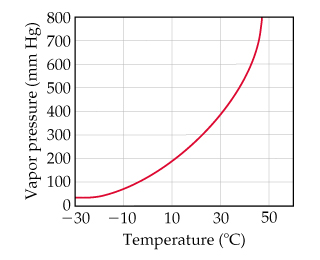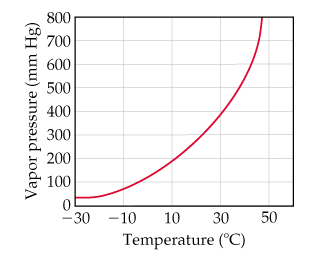# Problem: Using the graph,determine the following.The approximate vapor pressure of CS2 at 30 oC.

###### FREE Expert Solution

We are asked to approximate the vapor pressure of CS2 at 30°C using the graph below:###### Problem Details

Using the graph,

determine the following.

The approximate vapor pressure of CS2 at 30 oC.